# NCERT Solutions for Class 8 Maths Chapter 8 Comparing Quantities Ex 8.2

NCERT Solutions for Class 8 Maths Chapter 8 Comparing Quantities Exercise 8.2

Ex 8.2 Class 8 Maths Question 1.
A man got a 10% increase in his salary. If his new salary is ₹ 1,54,000, find his original salary.
Solution:
The increased salary = ₹ 1,54,000
Increase in salary = 10%
Increase salary = Original salary × (1 + $$\frac { Increase }{ 100 }$$)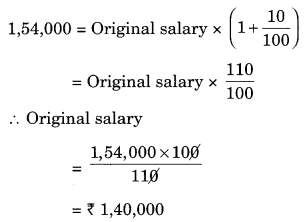Hence, the original salary = ₹ 1,40,000

Ex 8.2 Class 8 Maths Question 2.
On Sunday 845 people went to the Zoo. On Monday only 169 people went. What is the per cent decrease in the people visiting the Zoo on Monday?
Solution:
Number of people visiting the Zoo on Sunday = 845
Number of people visiting the Zoo on Monday = 169
Decrease in number of people visiting the Zoo = 845 – 169 = 676
Decrease per cent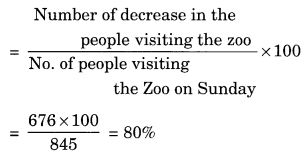Hence, the decrease per cent = 80%

Ex 8.2 Class 8 Maths Question 3.
A shopkeeper buys 80 articles for ₹ 2,400 and sells them for a profit of 16%. Find the selling price of one article.
Solution:
Cost price of 80 articles = ₹ 2,400
Cost of 1 article = ₹ $$\frac { 2400 }{ 80 }$$ = ₹ 30
Profit = 16%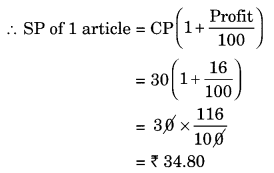Hence, the selling price of one article = ₹ 34.80

Ex 8.2 Class 8 Maths Question 4.
The cost of an article was ₹ 15,500. ₹ 450 were spent on its repairs. If it is sold for a profit of 15%, find the selling price of the article.
Solution:
CP of the article = ₹ 15,500
Money spent on repairs = ₹ 450
Net CP = ₹ 15,500 + ₹ 450 = ₹ 15,950
Profit = 15%Hence, the selling price of article = ₹ 18342.50

Ex 8.2 Class 8 Maths Question 5.
A VCR and TV were bought for ₹ 8,000 each. The shopkeeper made a loss of 4% on the VCR and a profit of 8% on the TV. Find the gain or loss per cent on the whole transaction.Solution:
Cost price of a VCR = ₹ 8,000
Loss = 4%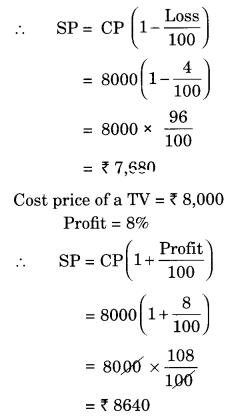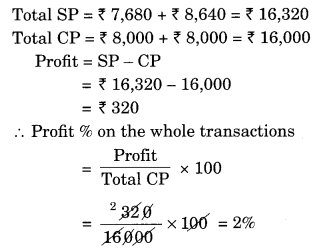Hence, the shopkeeper gained 2% profit on the whole transaction.

Ex 8.2 Class 8 Maths Question 6.
During a sale, a shop offered a discount of 10% on the marked prices of all the items. What would a customer have to pay for a pair of Jeans marked at ₹ 1450 and two shirts marked at ₹ 850 each?
Solution:
Marked Price (MP) of Jeans = ₹ 1,450
MP of two shirts = ₹ 850 × 2 = ₹ 1,700
Total MP = ₹ 1,450 + ₹ 1,700 = ₹ 3,150
Discount = 10%Thus, the customer will have to pay ₹ 2,835.

Ex 8.2 Class 8 Maths Question 7.
A milkman sold two of his buffaloes for ₹ 20,000 each. On one he made a gain of 5% and on the other a loss of 10%. Find his overall gain or loss. [Hint: Find CP of each]Solution:
SP of a buffalo = ₹ 20,000
Gain = 5%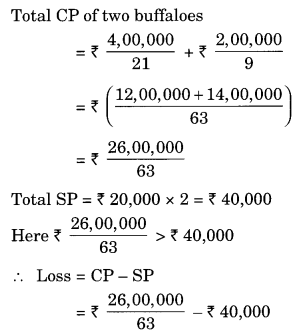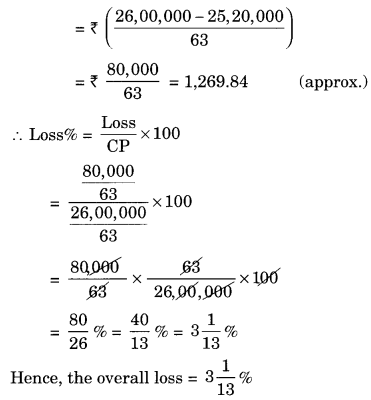Ex 8.2 Class 8 Maths Question 8.
The price of a TV is ₹ 13,000. The sales tax charged on it is at the rate of 12%. Find the amount that Vinod will have to pay if he buys it.
Solution:
Marked price of the TV = ₹ 13,000
ST = 12%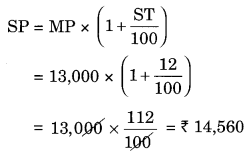The required amount that Vinod has to pay = ₹ 14,560

Ex 8.2 Class 8 Maths Question 9.
Arun bought a pair of skates at a sale where the discount is given was 20%. If the amount he pays is ₹ 1,600, find the marked price.
Solution:
Let the MP of the skates be ₹ 100
Discount = ₹ 20% of 100 = ₹ 20
Sale price = ₹ 100 – ₹ 20 = ₹ 80
If SP is ₹ 80 then MP = ₹ 100
If SP is ₹ 1 then MP = ₹ $$\frac { 100 }{ 80 }$$
If SP is ₹ 1,600 then MP = ₹ $$\frac { 100 }{ 80 }$$ × 1600 = ₹ 2,000
Thus the MP = ₹ 2000.

Ex 8.2 Class 8 Maths Question 10.
I purchased a hair-dryer for ₹ 5,400 including 8% VAT. Find the price before VAT was added.
Solution:
Let the original price be ₹ 100
VAT = 8% of 100 = ₹ 8
Sale price = ₹ 100 + ₹ 8 = ₹ 108
If SP is ₹ 108 then original price = ₹ 100
If SP is ₹ 1 then the original price = ₹ $$\frac { 100 }{ 108 }$$
If SP is ₹ 5,400 then the original price = ₹ $$\frac { 100 }{ 108 }$$ × 5,400 = ₹ 5,000
Thus, the price of hair-dryer before the addition of VAT = ₹ 5000.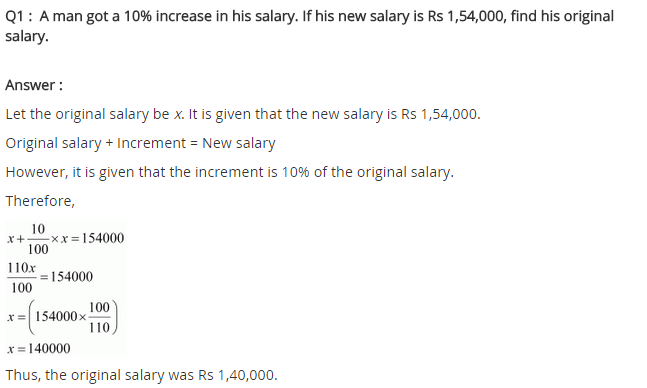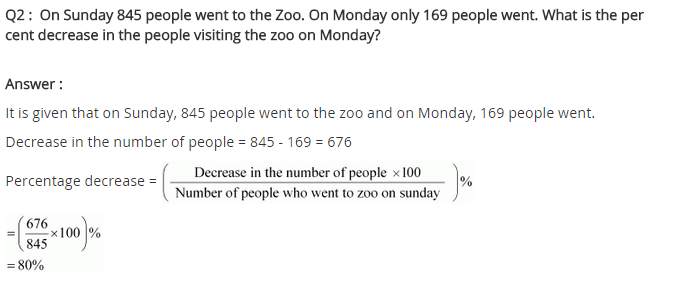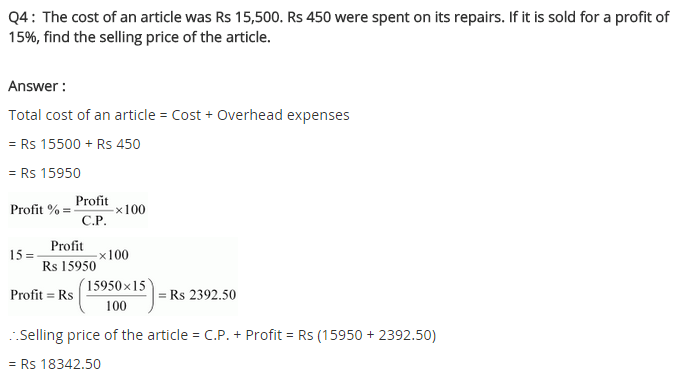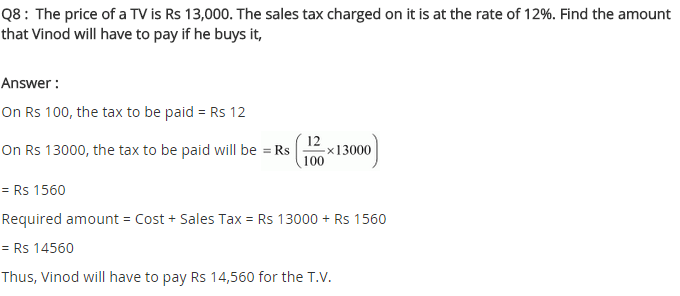+